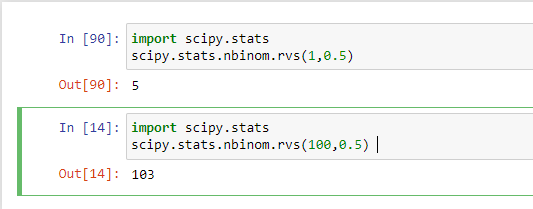# Generating random numbers from a probability distribution using SciPy's random moduleby sneha@openweaver.comUpdated: Mar 29, 2023Solution Kit

Generating random numbers from a probability distribution is a process of randomly selecting values from a set of data according to a probability distribution. A probability distribution is a mathematical function describing a random variable's likelihood of taking on a certain value.

Scipy is a free, open-source Python library for scientific and technical computing. It contains modules for optimization, signal and image processing, linear algebra, integration, interpolation, ODE solvers, special functions, FFT, and other tasks common in science and engineering.

The SciPy random module is a sub-package of SciPy, a collection of mathematical algorithms and convenience functions built on the NumPy extension of Python.

• It provides a suite of tools for generating random numbers, random sequences, and random variables with various probability distributions.
• It also includes functions for generating random numbers from various probability distributions, random permutations, and random sampling from a population.

Here is an example of generating random numbers from a probability distribution using SciPy's random module.Fig 1: Preview of the output that you will get on running this code from your IDE

### Code

In this solution, we use the SciPy stats module.

### Instructions

1. Install Jupyter Notebook on your computer.
2. Open terminal and install the required libraries with following commands.
3. Install SciPy - pip install scipy
4. Copy the snippet using the 'copy' button and paste it into that file.
5. Run the file using run button.

I hope you found this useful. I have added the link to dependent libraries, version information in the following sections.

I found this code snippet by searching for "Generating random numbers from a probability distribution using SciPy's random module" in kandi. You can try any such use case!

### Dependent Libraries

scipyby scipy

Python11340Version:v1.11.0rc1SciPy library main repository

Support
Quality
Security
Reuse

scipyby scipy

Python11340Version:v1.11.0rc1License: Permissive (BSD-3-Clause)

SciPy library main repository
Support
Quality
Security
Reuse

If you do not have SciPy which is required to run this code, you can install it by clicking on the above link and copying the pip Install command from the SciPy page in kandi.

You can search for any dependent library on kandi like SciPy.

### Environment Tested

I tested this solution in the following versions. Be mindful of changes when working with other versions.

1. The solution is created in Python3.9.6
2. The solution is tested on SciPy-Python 1.9.1 version.

Using this solution, we are able to generate random numbers from a probability distribution using SciPy's random module.

This process also facilities an easy to use, hassle free method to create a hands-on working version of code which would help us to generate random numbers from a probability distribution in Python

### Support

1. For any support on kandi solution kits, please use the chat
2. For further learning resources, visit the Open Weaver Community learning page.

See similar Kits and Libraries